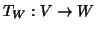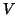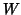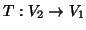Next: RMS Deviation Up: tr99mj1 Previous: Introduction

# Transformations

A transformation from one volume to another can act either in voxel coordinates, world coordinates or a combination of both. In general, voxel and world coordinates are related by a simple affine transformation, whereis the voxel coordinate space, andthe world coordinate space. By using this transformation, all transformations can be expressed as transformations between world coordinates, which is more convenient and will be assumed from here on.

For example, ifis a transformation between two voxel coordinate spaces, thenis the corresponding transformation between the world coordinate spaces.

Mark Jenkinson 2003-02-11